• python如何保留小数点位数

千次阅读 2021-04-26 19:29:51
python保留小数点位数的方法：首先新建py文件，输入【a=('%.2f' % a)】即可保留2位小数；然后如果输入【a=('%.4f' % a)】，就保留4位小数；**后也可以输入【a=format(a, '.2f')】来保留小数点位数。0Z8少儿编程网-...

python保留小数点位数的方法：首先新建py文件，输入【a=('%.2f' % a)】即可保留2位小数；然后如果输入【a=('%.4f' % a)】，就保留4位小数；**后也可以输入【a=format(a, '.2f')】来保留小数点位数。0Z8少儿编程网-https://www.pxcodes.com0Z8少儿编程网-https://www.pxcodes.com

本教程操作环境：windows7系统、pycharm2020版，DELL G3电脑。0Z8少儿编程网-https://www.pxcodes.com

python保留小数点位数的方法：0Z8少儿编程网-https://www.pxcodes.com

第一步、打开pycharm，新建一个py文件，输入0Z8少儿编程网-https://www.pxcodes.coma=1.2222345

a=('%.2f' % a)

print(a)

代码，如下图所示：

0Z8少儿编程网-https://www.pxcodes.com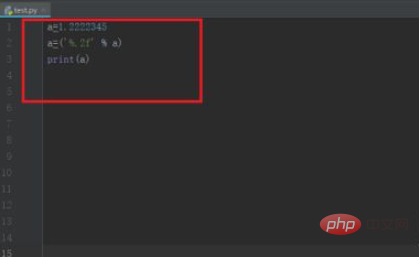0Z8少儿编程网-https://www.pxcodes.com

第二步、运行py文件之后，可以看到a小数点保留2位小数了，如下图所示：0Z8少儿编程网-https://www.pxcodes.com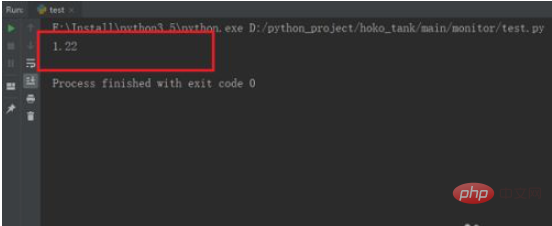0Z8少儿编程网-https://www.pxcodes.com

第三步、如果输入a=('%.4f' % a)，就保留4位小数，需要注意是四舍五入，如下图所示：0Z8少儿编程网-https://www.pxcodes.com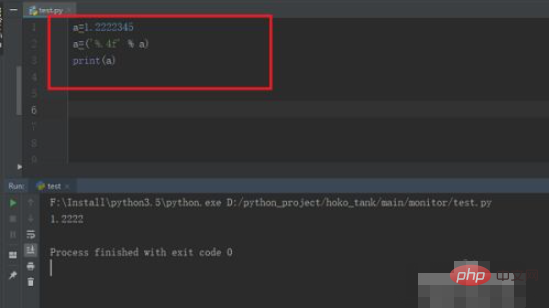0Z8少儿编程网-https://www.pxcodes.com

第四步、我们也可以输入a=format(a, '.2f')来保留小数点位数，如下图所示：0Z8少儿编程网-https://www.pxcodes.com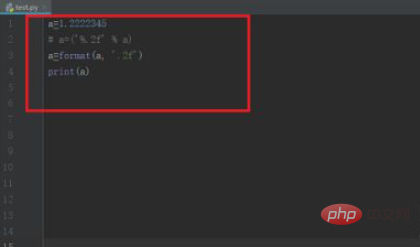0Z8少儿编程网-https://www.pxcodes.com

第五步、运行py文件，可以看到保留2位小数，同样也是四舍五入，如下图所示：0Z8少儿编程网-https://www.pxcodes.com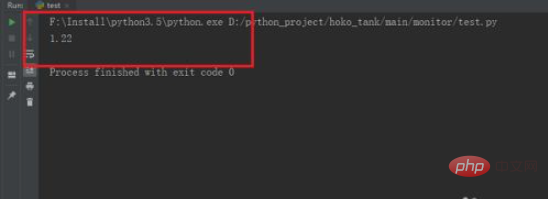0Z8少儿编程网-https://www.pxcodes.com相关免费学习推荐：python视频教程0Z8少儿编程网-https://www.pxcodes.com

展开全文• C++中保留小数点位数： 需包含头文件 #include<iomanip> io代表输入输出，manip是manipulator 需要包含语句 cout << setiosflags(ios::fixed) << setprecision(n); 例题： 华氏度转换为...

C++中保留小数点后位数

• 需包含头文件
#include<iomanip>

io代表输入输出，manip是manipulator

• 需要包含语句
cout << setiosflags(ios::fixed) << setprecision(n);

例题：
华氏度转换为摄氏度：

#include<iostream>
#include<iomanip>
using namespace std;
int main()
{
double F;
while (cin >> F)
{
cout << setiosflags(ios::fixed) << setprecision(2);
cout <<"c="<< 5 * (F - 32) / 9 << endl;
}
return 0;
}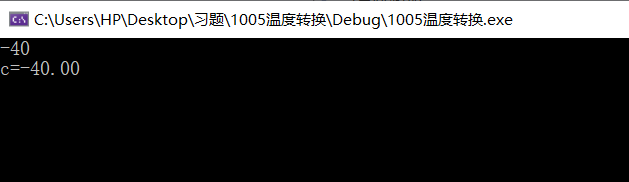展开全文• java保留两位小数问题：方式一：四舍五入doublef=111231.5585;BigDecimalb=newBigDecimal(f);doublef1=b.setScale(2,BigDecimal.ROUND_HALF_UP).doubleValue();保留两位小数---------------------------------------...

java保留两位小数问题：

方式一：

四舍五入 double f = 111231.5585; BigDecimal b = new BigDecimal(f); double f1 = b.setScale(2, BigDecimal.ROUND_HALF_UP).doubleValue(); 保留两位小数 ---------------------------------------------------------------

方式二：

java.text.DecimalFormat df =new java.text.DecimalFormat("#.00"); df.format(你要格式化的数字);

例：new java.text.DecimalFormat("#.00").format(3.1415926)

#.00 表示两位小数 #.0000四位小数 以此类推...

方式三：

double d = 3.1415926;

String result = String .format("%.2f",d);

%.2f %. 表示 小数点前任意位数 2 表示两位小数

格式后的结果为f 表示浮点型

方式四：

NumberFormat ddf1=NumberFormat.getNumberInstance() ;

void setMaximumFractionDigits(int digits)

digits 显示的数字位数

为格式化对象设定小数点后的显示的最多位,显示的最后位是舍入的

import java.text.* ;

import java.math.* ;

class TT

{

public static void main(String args[])

{ double x=23.5455;

NumberFormat ddf1=NumberFormat.getNumberInstance() ;

ddf1.setMaximumFractionDigits(2);

String s= ddf1.format(x) ;

System.out.print(s);

}

}

展开全文java 小数点位数
• 第一种方法-使用DecimalFormat类举个例子，假如我们需要保留两位小数，我们可以这样写DecimalFormat df = new DecimalFormat("0.00");测试如下：double d = 0.200;DecimalFormat df = new DecimalFormat("0.00");...

第一种方法-使用DecimalFormat类

举个例子，假如我们需要保留两位小数，我们可以这样写

DecimalFormat df = new DecimalFormat("0.00");

测试如下：

double d = 0.200;

DecimalFormat df = new DecimalFormat("0.00");

System.out.println(df.format(d));

输出结果为：

0.20

若double d=0.000；输出结果为0.00；

若double d=0；输出结果为0.00；

若double d=41.2345；输出结果为41.23；

经测试，不管double d的值为多少，最后结果都是正常的两位小数。

同理若是保留一位小数DecimalFormat df = new DecimalFormat("0.0");其他以此类推

若是这种写法DecimalFormat df = new DecimalFormat("0.00")，不管传入的任何值，均保留两位小数

还有一种写法是这样：

double d = 41.123;

DecimalFormat df = new DecimalFormat("#.##");

System.out.println(df.format(d));

输出结果为：

41.12

若double d=2.00，输出结果为2；

若double d=41.001，输出结果为41；

若double d=41.010，输出结果为41.01；

若double d=0，输出结果为0；

若double d=0.200，输出结果为0.2；

总结：若是这种写法DecimalFormat df = new DecimalFormat("#.##")，则保留小数点后面不为0的两位小数，这种写法不能保证保留2为小数，但能保证最后一位数不为0；

第二中方法

测试：

double d = 0.6544;

String s=String.format("%.2f",d);

System.out.println(s);

输出结果为：

0.65

若double d=0.6566，输出结果为0.66；

若double d=0，输出结果为0.00;

其中String s=String.format("%.2f",d)表示小数点后任意两位小数，其中2为表示两位小数，若需要三位小数，把2改为3即可，其他同理。

总结：这种方法不管传入的值是多少，均保留两位小数，并且符合四舍五入的规则。

第三种方法-使用BigDecimal类

测试：

double d = 1.000;

BigDecimal bd=new BigDecimal(d);

double d1=bd.setScale(2,BigDecimal.ROUND_HALF_UP).doubleValue();

System.out.println(d1);

输入结果:1.0

若double d=0，输出结果为0.0；

若double d=1.999，输出结果为2.0；

若double d=1.89，输出结果为1.89；

总结：使用这种写法若小数点后均为零，则保留一位小数，并且有四舍五入的规则。

源码

以下为Java测试类

import java.math.BigDecimal;

import java.text.DecimalFormat;

public class Test {

public static void main(String[] args) {

double d = 1.19;

System.out.println(formatDecimal4(d));

}

public static String formatDecimal1(double d) {

DecimalFormat df = new DecimalFormat("0.00");

return df.format(d);

}

public static String formatDecimal2(double d) {

DecimalFormat df = new DecimalFormat("#.##");

return df.format(d);

}

public static String formatDecimal3(double d){

return String.format("%.2f",d);

}

public static double formatDecimal4(double d){

BigDecimal bd=new BigDecimal(d);

double d1=bd.setScale(2,BigDecimal.ROUND_HALF_UP).doubleValue();

return d1;

}

}

展开全文java 小数点保留
• Python：使用f-string保留小数点位数 格式 f"{num:xxx}" 其中xxx的格式如下 格式 说明 width 整数width指定宽度 0width 整数width指定宽度，0表示最高位用0补足宽度 width.precision 整数width指定...
• 有时候我们在时候java的时候需要保留小数点位数，那么你知道java代码如何保留小数点位数吗？那么下面就让爱站小编为大家介绍java代码保留小数点位数实现方法。import java.text.* ;import java.math.* ;public...
• '.5f')) 结果：1.23334 3、round()函数 round()函数和format函数一样，也是可以四舍五入的，但是这个函数有好多坑，不建议使用 同样时保留小数点后五位 a = 1.23333644022 print(round(a, 5)) 结果：1.23334 4、...
• 1.创建测试表SQL> create table t_int(id number(20,3),name varchar2(10));Table created.SQL> insert into t_int values(3.1456,'a');1 row created.SQL> select *from t_int;ID NAME---------- --------...
• 前面的数，表示了即将输出的这个数占多少位数，.**后面的数，表示了即将输出的这个数将保留多少位小数 同时，如果 . 前面的数是一个正数，则说明它是向右对齐的，即补位补在前面，如果是负数，则相反。
• 如何设置python的小数点保留位数 如 a=1.234567 a=('%.2f' % a) print(a) 即保留小数点后两位 同理若将a=(’%.2f’ % a)更换为a=(’%.4f’ % a) 即保留小数点后四位。以此类推。
• 计算器怎么改小数点位数

千次阅读 2021-06-19 02:07:50
模拟出的108级数据都是一样的但是实际2只宠数据是有点小差别的，血魔跟模拟数字都差了2点影响不大，但只能说是基本准确这个问题我也遇见过但是不知道是怎么回事~~你可以去问问暗月吧!! 都是2攻问题很简单，小数点的...
• c#保留小数点后位数的方法

千次阅读 2020-12-22 18:22:03
Double dValue = 95.12345;int iValue = 10000;string strValue = "95.12345";string result = "";result = Convert.ToDouble(dValue)....//保留小数点后两位,结果为95.12result = Convert.ToDouble(iValue).ToSt...
• 本节内容：number_format()保留小数点后几位PHP保留小数点后2位的函数number_format：number_format(带小数点的书,小数点保留位数)例子：复制代码 代码示例:number_format(8.3486,2); //取得小数点后2位有效数//...php保留小数位数
• 在C++中输出要控制小数点位数需要用到头文件 iomanip 中的 setprecision(n) 设置精度 和 setiosflags(ios::fixed)其中setprecision(n)设置浮点数的有效数字为nsetiosflags(ios::fixed)设置浮点数以固定的小数位数...
• 保留两位小数方法一：{double c = 3.154215;java.text.DecimalFormat myformat=new java.text.DecimalFormat("0.00");String str = myformat.format(c);}方式二：{java.text.DecimalFormat df = new java.text....
• 今天遇到个需要将一个double类型的数据保留小数点后两位的问题。在网上搜寻的集中解决方案,copy来一下,备用首先result_value是处理前的doubleget_double是用来保存我处理后的double//方案一:get_double = (double)...
• 今天项目中需要更改时长的显示方式，规定必须保留两位小数，刚才看简书的时候正好看到一个指定保留小数位数的工具类的文章，在此基础上，做了一点小修改，用起来更加方便了，有需要的朋友尽管撸走DecimalUtils 类：...
• Python 如何强制限定小数点位数利用''%.af''%b——其中 b 代表要限定的数字， a 代表要求限定小数点的位数，结果自动四舍五入。例：c = 1.264871331241212print("%.3f"%c)运行结果：1.265补充：Python Numpy数组格式...
• Python如何取小数点位数

千次阅读 2021-01-13 06:39:53
四舍五入，保留几位小数 from decimal import * Decimal(‘50.5679‘).quantize(Decimal(‘0.00‘)) # 结果为Decimal(‘50.57‘)，结果四舍五入保留了两位小数 5.Decimal 结果转化为string from decimal import * ...
• Python之路-jQuery 参考网址:... js基础知识：字面量 关键字和保留字 js中的字面量概念我的理解就是:字体表面的常量 如:数字 100, 字符串 "ssss"或'ssss' 布尔值:false ,正则 以及null对象. 这些都是常量. 关键字: ...
• 0和#都是占位符，但在不同的地方，作用不一样0：比实际数字的位数多，不足的地方用0补上。new DecimalFormat("00.00").format(3.14) //结果：03.14new DecimalFormat("0.000").format(3.14) //结果： 3.140new ...
• 1.取四舍五入的几位小数selectround(1.2345,3)fromdual;...保留两位小数，只舍selecttrunc(1.2345,2)fromdual;结果:1.23selecttrunc(1.2399,2)fromdual;结果:1.233.取整数返回大于或等于x的最大整数:SQL>selectc...
• - HTML小数点限制两位小数：正整数：var cont={init: function(){this.item_price();},item_price: function() {amountInput("#itemForm",".js_type");//限定为正整数\$("#itemForm").on("keyup",".js_stock",...
• 例如: 保留小数点后1位，则 n = 10 * n= 1 / 0.1 保留小数点后2位，则 n = 100 * n= 1 / 0.01 保留小数点后3位，则 n = 1000 * n= 1 / 0.001 至于为什么是先乘后除，可以参考如下文章： ...
• 输入a b n输出一行数字样例输入97 61 50样例输出1.59016393442622950819672131147540983606557377049180就是有些测试点过不了，大神们帮我看看，我有何遗漏的地方我的程序：考虑了小数点为0考虑了最后进位，包括进位...
• MySQL查询小数点位数

千次阅读 2021-01-19 15:16:14
怎么查询某个字段中小数有几位？MySQL数据库：通过下面sql就可以查出来，有2位col*100，有3位col*1000，一次类推；select * from ws_inventory_item where real_quantity*1000 - floor(real_quantity*1000) > 0...
• 关于处理小数点位数的几个oracle函数... 保留两位小数，关于处理小数点位数的几个Oracle函数()1. 取四舍五入的几位小数select round(1.2345, 3) from dual;结果:1.2352. 保留两位小数，只舍select trunc(1.2345, 2) ...
• 在C的编程中，总会遇到浮2113点数的处理，有5261的时候，我们只需要保留2位小数4102作为输出的结果，这时候，1653问题来了，怎样才能让cout输出指定的小数点保留位数呢？可以通过printf函数中的输出格式说明符来......